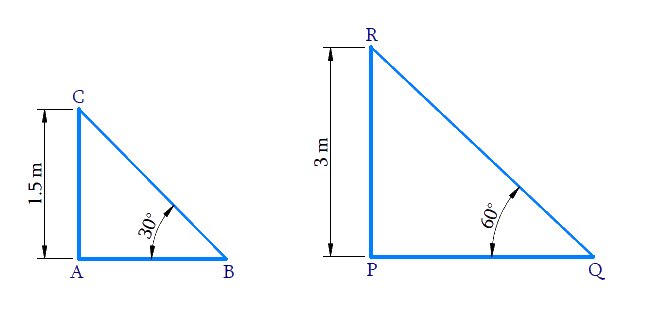# Ex.9.1 Q3 Some Applications of Trigonometry Solution - NCERT Maths Class 10

Go back to  'Ex.9.1'

## Question

A contractor plans to install two slides for the children to play in a park. For the children below the age of $$5$$ years, she prefers to have a slide whose top is at a height of $$1.5\,\rm{m}$$ and is inclined at an angle of $$30^\circ$$ to the ground, whereas for elder children she wants to have a steep slide at a height of $$3\,\rm{m}$$ and inclined at an angle of $$60^\circ$$ to the ground. What should be the length of the slide in each case?

Video Solution
Some Applications Of Trigonometry
Ex 9.1 | Question 3

## Text Solution

What is Known?

(i) For the children below the age of $$5$$ years.

Height of the slide $$=$$ $$1.5\,\rm{m}$$

Slide’s angle with the ground $$=$$ $$30^\circ$$

What is Unknown?

Length of the slide for children below the age of $$5$$ years and elder children.(ii) For elder children.

Height of the slide $$= 3\,\rm{m}$$

Slide’s angle with the ground $$=$$$$60^\circ$$

Reasoning:

Let us consider the following conventions for the slide installed for children below $$5$$ years :

• The height of the slide as $$AC.$$
• Distance between the foot of the slide to the point where it touches the ground as $$AB.$$
• Length of the slide as $$BC.$$

Let us consider the following conventions for the slide installed for elder children:

• The height of the slide $$PR.$$
• Distance between the foot of the slide to the point where it touches the ground as $$PQ.$$
• Length of the slide as $$QR.$$

(i) Trigonometric ratio involving $$AC, BC$$ $$\angle B$$ and is $$\sin \theta$$

(ii) Trigonometric ratio involving $$PR, QR$$ and $$\angle Q$$ and is $$\sin \theta$$

Steps:

(i) In $$\Delta ABC$$,

\begin{align} \sin 30^{\circ} &=\frac{A C}{B C} \\ \frac{1}{2} &=\frac{1.5}{B C} \\ B C &=1.5 \times 2 \\ B C &=3 \mathrm{m}\end{align}

(ii) In $$\Delta \rm{PRQ}$$,

\begin{align}\sin \,Q &= \frac{{PR}}{{QR}}\\\sin \,60^\circ & = \frac{3}{{QR}}\\\frac{{\sqrt 3 }}{2}& = \frac{3}{{QR}}\\QR &= \frac{{3 \times 2}}{{\sqrt 3 }}\\&= \frac{6}{{\sqrt 3 }} \times \frac{{\sqrt 3 }}{{\sqrt 3 }}\\&= \frac{{6\sqrt 3 }}{3}\,\\&= 2\sqrt 3 \end{align}

Length of slide for children below $$5$$ years $$= 3\rm{m}.$$

Length of slide for elder children $$=$$ $$2 \sqrt3\,\rm{m}$$

Learn from the best math teachers and top your exams

• Live one on one classroom and doubt clearing
• Practice worksheets in and after class for conceptual clarity
• Personalized curriculum to keep up with school